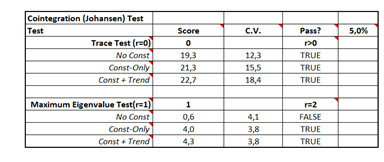# Cointegration in NumXL

## Question:

How do I know when cointegration exists in my results?According to the table above, for cointegration to exist you must have the following:

1. In the first part (upper) of the table, where we are testing r>0, This should be true.
2. In the 2nd part (lower) of the table, where were are testing r=2, This should be false.

Now, we have several test scenarios: no const, const, const + trend. For each scenario, we need to examine the cointegration assumptions.

Step 1: Look for the one that assumes nothing, we label this scenario “no const.” If no cointegration is present, proceed to step 2. Otherwise, stop.

Step 2: Look for the scenario that assumes a constant, we label this scenario “const.” If no cointegration is present, proceed to step 3. Otherwise, stop.

Step 3: Look for the scenario which assumes a constant and trend, we label this “const + trend.” If no cointegration then stop.